# Modal Class

Here we will learn about modal class, including what it is and how to find the modal class from a grouped frequency table.

There are also modal class worksheets based on Edexcel, AQA and OCR exam questions, along with further guidance on where to go next if you’re still stuck.

## What is modal class?

The modal class is the class interval within a set of data that contains the most number of data points, which we can view as the highest frequency

For example,
Below we have a set of data showing the marks achieved by 65 students in a test. The class interval with the most number of data points is ‘21 to 30’ as this class interval has the highest frequency of 16 (the class interval contains 16 items of data). We would therefore state,

The modal class is 21 to 30 .

### What is modal class?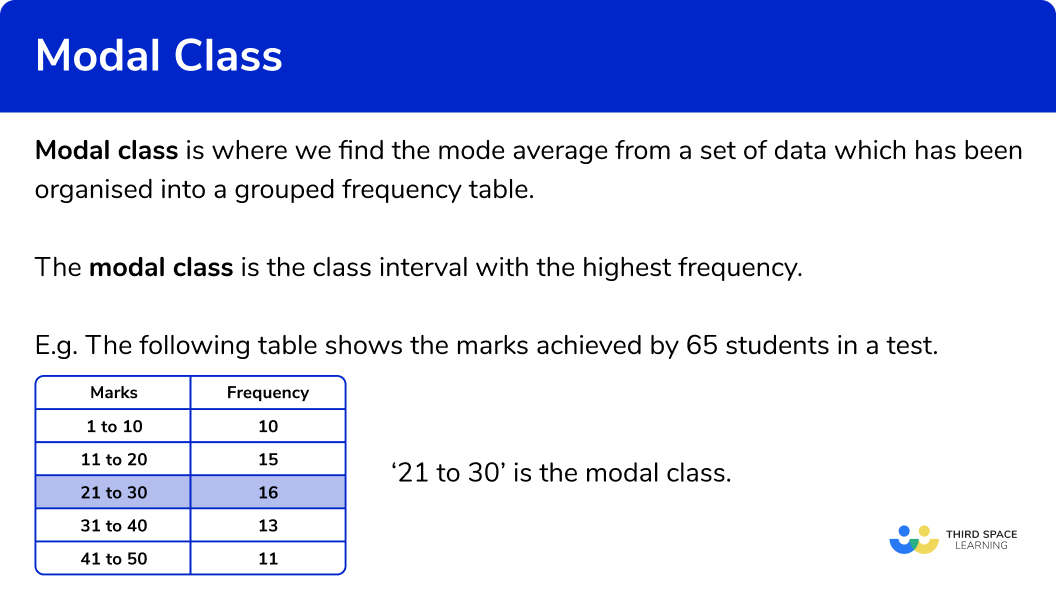## Modal class from a graph / chart

Sometimes you may be given a bar chart, a pie chart, a frequency polygon, a cumulative frequency curve, or even a histogram, and be asked to state the modal class from this graph. You, therefore, need to be able to interpret the data from each graph.

Below is a table summarising how to state the modal class from common types of graph/chart.

## How to find the modal class

In order to find the modal class:

1. Look for the class interval with the highest frequency.
2. Write down the modal class.

### How to find the modal class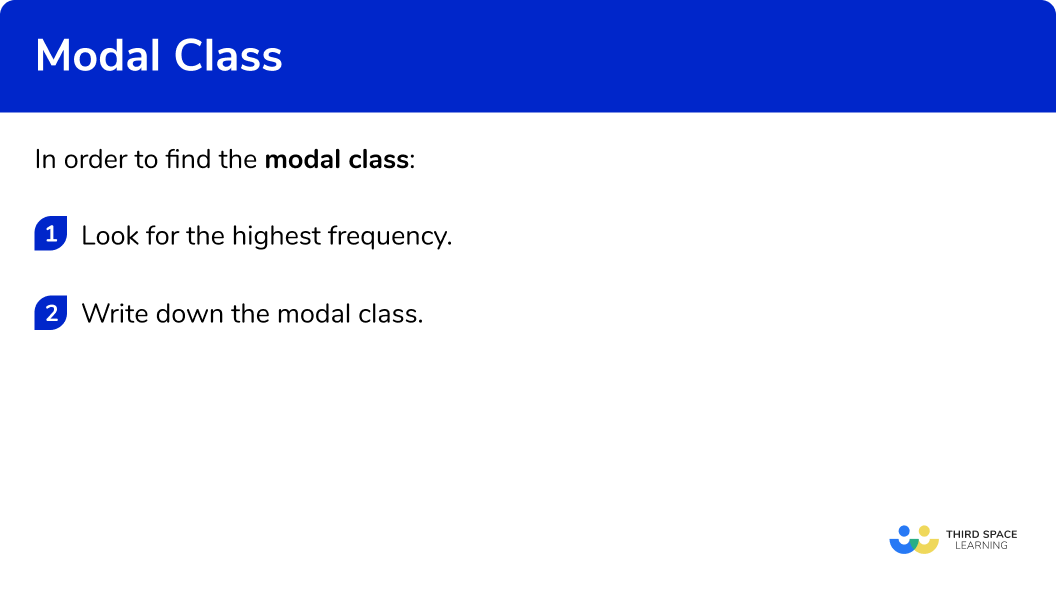## Related lessons on frequency tables

Modal class is part of our series of lessons to support revision on frequency tables. You may find it helpful to start with the main frequency table lesson for a summary of what to expect, or use the step by step guides below for further detail on individual topics. Other lessons in this series include:

## Modal class examples

### Example 1: modal class from a frequency table

The grouped frequency table shows the number of people in 80 shops in a town. State the modal class from the following table.

1. Look for the class interval with the highest frequency.

The highest frequency is 26

2Write down the modal class.

The class with the highest frequency is ‘18 to 21’ .

The modal class is ‘18 to 21’ .

### Example 2: modal class from a frequency table

The grouped frequency table shows the number of pupils in 90 primary schools in an area. State the modal class from the following set of data.

The highest frequency is 23 .

The associated class with the highest frequency is ‘100 - 149’ .

The modal class is ‘100 - 149’ .

### Example 3: modal class from a frequency table

The grouped frequency table shows the number of marks achieved by 100 students in their science test. State the modal class from the results table below.

The highest frequency is 37 .

The associated class with the highest frequency is '40\le x<60' .

The modal class is '40\le x<60' .

## How to find the modal class from a graph / chart

In order to find the modal class from a graph / chart:

1. Look for the specific feature for the mode on the graph/chart.
2. Write down the modal class.

## Modal class from a graph or chart examples

### Example 4: modal class from a bar chart

The bar chart below shows the frequencies of pet ownership from a group of people. Each person owns one pet. State the modal class from the bar chart below.

The category with the highest frequency is ‘Cat’.

The modal class is Cat.

### Example 5: modal class from a cumulative frequency diagram

30 students ran 75m . Their time was recorded in a cumulative frequency diagram. Using the information below, determine the modal class for the set of data.

As we have a cumulative frequency table, the class with the highest frequency will have the largest increase from one time to the next.

Using the table, we have the largest increase between 12 and 14 seconds.

Also, on the cumulative frequency diagram, this class interval has the steepest gradient:

As this group represents the time above 12 seconds and up to 14 seconds we have the modal class interval to be 12 < s \le 14 .

### Example 6: modal class from a histogram

The histogram below represents the distance a ball was thrown by 40 people. State the frequency of the modal class.

The class interval for a histogram with the highest frequency has the largest area (as the area of each bar is the frequency of the class).

The modal class is 15 \le x < 20 .

### Common misconceptions

• Check which average you are being asked for

Check if you have been asked for the median, mode (modal class) or mean average.

• Multiple modes

A data set can have 1 mode or 2 modes (bimodal). It is possible that a data set has no modal group at all.

• Item from the data set

The mode will be the item – NOT the frequency.

• Class intervals notation

The class intervals used in grouped frequency tables can be written in different ways. Take care when writing them, or finding the midpoints.

For example,

0 to 5

0-5

0\le x <5

• Highest frequency

A number may appear more than once in a frequency column. This does NOT affect the modal class. The modal class is always indicated by the largest frequency, not the frequency that occurs the most often.

For example,

The modal class is ‘20 to 29’ as this interval has the highest frequency. (We do not need to consider the fact that the frequency ‘5’ appears twice in the frequency column.)

### Practice modal class questions

1. The table shows the grouped data about the scores in 35 netball matches. State the modal class interval.131220 to 2910 to 19The highest frequency is 13 . The associated class is ‘10 to 19’ . So the modal class is ‘10 to 19’ .

2. The table shows the grouped data about the number of eggs laid by 50 chickens in two weeks. State the modal class interval.1812-146-89-11The highest frequency is 18 . The associated item is ‘6-8’ . So the modal class is ‘6-8’ .

3. The table shows the grouped data about the height of 100 trees in a forest.
State the modal class interval.15 < h \le203120 < h \le2524The highest frequency is 31 . The associated item is ‘15 < h \le20’ . So the modal class is ‘15 < h \le20’ .

4. The pie chart below shows the number of different types of sweets in a jar. State the modal class for the pie chart.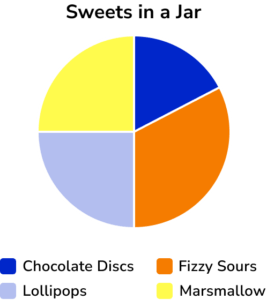Chocolate DiscsFizzy SoursLollipops and MarshmallowsLollipopsThe largest segment of the circle is orange, which represents fizzy sours. (Angle 120^{\circ} ).

5. Data was recorded for the speed of a number of 100 vehicles. Each class interval is the same size. Use the frequency polygon below to determine the modal class for the set of data.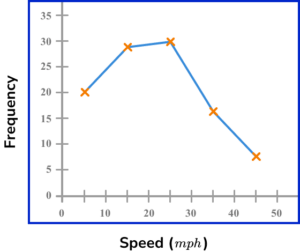15\le x < 3510\le x < 2020\le x < 3035\le x < 45A frequency polygon plots the frequency of the group against the midpoint of the class interval.

As the class intervals are the same size, and the distance between each midpoint is 10mph , each class interval is 10mph . The upper bound for each class is 5mph above the midpoint, and the lower bound is 5mph lower than the midpoint.

The highest frequency is the mode and so the highest plotted value is at (25,30) , so the modal class interval is 20\le x<30 .

6. The histogram below represents the data collected for a recent score on a road safety test.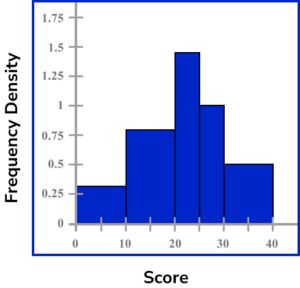Determine the modal class of the data.

10\le x < 2025\le x < 3030\le x < 4020\le x < 25The modal class has the largest area. The area of each class is,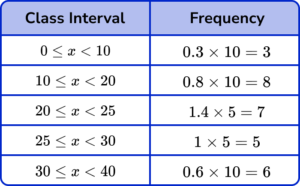The class interval with the largest area / highest frequency is 10\le x < 20 .

### Modal class GCSE questions

1. The table shows the speed, x of 140 vehicles on a motorway.State the modal class interval.

(1 mark)

65\le x<70

(1)

2. The table shows the time 1427 people spend in a gym during one day.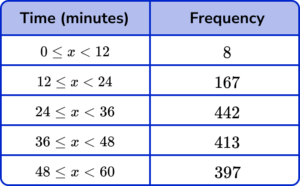State the modal class interval.

(1 mark)

24\le x<36

(1)

3. The table shows the number of goals scored in 22 matches.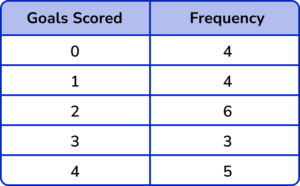Justin says “the modal class is 4 because it occurs the most often”. Is Justin correct? Explain your answer.

(2 marks)

No

(1)

The modal class is 2 because it has the highest frequency.

(1)

## Learning checklist

You have now learned how to:

• Interpret data sets from univariate empirical distributions through appropriate measures of central tendency (including modal class)

## Still stuck?

Prepare your KS4 students for maths GCSEs success with Third Space Learning. Weekly online one to one GCSE maths revision lessons delivered by expert maths tutors.

Find out more about our GCSE maths tuition programme.